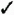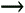# Section 52. About Calc FunctionsBEFORE YOU BEGINSEE ALSO

53 Enter Calc Functions

Entering individual formulas can get tedious . Suppose you want to add all the values in a column of 100 cells . You would type a formula such as =F2+F3+F4+ and would likely run out of roomand patience!before you complete the formula. In addition, such long formulas are likely to produce errors when you have to type so much.

Fortunately, Calc includes several built-in functions that perform many common mathematical calculations. Instead of writing a formula to sum a row or column of values, for example, you would use the Sum() function.

Function names always end with parentheses, such as Average() . A function accepts zero or more arguments . A function might use zero, one, or more arguments, depending on how much information the function needs to do its job. When using multiple arguments in a function, separate the arguments with semicolons. If a function contains only a single argument, do not use a semicolon inside the parentheses. Functions generally manipulate data ( numbers or text), and the arguments inside the parentheses supply the data to the functions. The Average() function, for example, computes an average of whatever list of values you pass in the argument. Therefore, all the following compute an average from the argument list:

`  =Average(18; 65; 299; \$R; 10; -2; 102)   =Average(SalesTotals)   =Average(D4:D14)  `KEY TERM

Arguments Values appearing inside a function's parentheses that the function uses in some way to produce its result.TIP

When you begin to enter a formula, ToolTips pop up after you start to type the formula's name to help guide you through the formula's required contents. When you type =A into a cell , a ToolTip pops up that reads =AVERAGE . You can take advantage of Calc's AutoComplete feature by pressing Enter when you see the ToolTip if that's the function you're entering and not another, such as =Abs() .

When you type a function name, whether you type it in uppercase or lowercase letters, Calc converts the name in your formula to all uppercase letters .

As with some functions, Average() accepts as many arguments as needed to do its job. The first Average() function computes the average of seven values, one of which is an absolute cell reference. The second Average() function computes the average of a range named SalesTotals . No matter how many cells comprise the range SalesTotals , Average() computes and displays the average. The final Average() function shows the average of the values in the range D4 through D14 (a columnar list).

Functions improve your accuracy. If you want to average three cell values, for example, you might type something such as this:

`  =C2 + C4 + C6 / 3  `

However, this formula does not compute an average! Remember that the operator hierarchy forces the division calculation first. If you use the Average() function, as shown next , you don't have to worry as much about the calculation's hierarchy:

`  =Average(C2; C4; C6)  `

The Sum() function is perhaps the most common function because you so often total columns and rows. Instead of adding each cell individually, you could more easily enter the following function:

`  =Sum(F2:F101)  `TIP

When you insert rows within the Sum() range, Calc updates the range inside the Sum() function to include the new values.

You can use functions inside other formulas. The following formula might be included in a cell that works on sales totals:

`  =CostOfSales * Sum(Qtr1; Qtr2; Qtr3; Qtr4) / SalesFactor * 1.07  `

Table 7.1 describes common built-in functions for which you'll find a lot of uses as you create spreadsheets. Remember to start every formula with an equal sign and to add your arguments to the parentheses, and you are set.

##### Table 7.1. Common Calc Functions

Function Name

Description

Abs()

Computes the absolute value of its cell argument. (Good for distance- and age-difference calculations.)

Average()

Computes the average of its arguments.

Count()

Returns the number of numerical arguments in the argument list. (Useful if you use a range name for the argument list.)

Max()

Returns the highest (maximum) value in the argument list. (Useful if you use a range name for the argument list and you need to pick out the highest value.)

Min()

Returns the lowest (minimum) value in the argument list. (Useful if you use a range name for the argument list and you need to pick out the lowest value.)

Pi()

Computes the value of mathematical pi (requires no arguments) for use in math calculations.

Product()

Computes the product (multiplicative result) of the argument range.

Roman()

Converts its cell value to a roman numeral.

Sqrt()

Computes the square root of the cell argument.

Stdev()

Computes the argument list's standard deviation.

Sum()

Computes the sum of its arguments.

Today()

Returns today's date (requires no arguments).

Var()

Computes a list's sample variance.NOTE

Calc supports many functions, including complex mathematical, date, time, financial, and engineering functions. Select Help to get more details on all the functions you can use.Sams Teach Yourself OpenOffice.org 2, Firefox and Thunderbird for Windows All in One
ISBN: 0672328089
EAN: 2147483647
Year: 2005
Pages: 232
Authors: Greg Perry